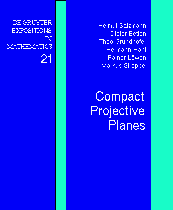H. Salzmann, D. Betten, T. Grundhöfer, H. Hähl, R. Löwen, M. Stroppel

## Compact Projective Planes

With an introduction to octonion geometry.
de Gruyter Expositions in Mathematics, vol 21, Berlin 1995.

## Table of Contents:

Preface
Table of contents
Chapter 1   The classical planes
11    The classical division algebras
12    The classical affine planes
13    The projective planes over R, C and H
14    The planes over R, C, and H as topological planes
15    Geometry of a projective line
16    The projective octonion plane P2O
17    The collineation group of P2O
18    Motion groups of P2O
Chapter 2   Background on planes, coordinates and collineations
21    Projective and affine planes
22    Coordinates, ternary fields
23    Collineations
24    Lenz-Barlotti types
25    Translation planes and quasifields
Chapter 3   Geometries on surfaces
31    R2-planes
32    Two-dimensional compact projective planes
33    Towards classification
34    The Moulton planes
35    Skew hyperbolic planes
36    Skew parabola planes
37    Planes over Cartesian fields
38    Flexibility, rigidity and related topics
Chapter 4   Compact projective planes
41    The topology of locally compact planes
42    Compact connected planes
43    Ternary fields for compact planes
44    Automorphism groups
Chapter 5   Algebraic topology of compact, connected planes
51    General properties
52    Assuming that lines are manifolds
53    Conditions implying that lines are manifolds
54    Lines are homology manifolds
55    Geometric consequences
Chapter 6   Homogeneity
61    Axial collineations
62    Planes admitting a classical motion group
63    Transitive groups
64    Transitive axial groups
65    Groups of large dimensions
66    Remarks on von Staudt's point of view
Chapter 7   Four-dimensional planes
71    Automorphism groups
72    Characterizing P2C
73    Four-dimensional translation planes
74    Four-dimensional shift planes
75    Analytic planes
Chapter 8   Eight- and sixteen-dimensional planes
81    Translation planes
82    Classification of translation planes
83    Stiffness
84    Characterizing P2H
85    Characterizing P2O
86    Hughes planes
87    Principles of classification
Chapter 9   Appendix: Tools from topology and Lie theory
91    Permutation groups
92    Topological dimension and remarks on general topology
93    Locally compact groups and Lie groups
94    Lie groups and their structure
95    Linear representations
96    Transformation groups
Bibliography
Notation
Index